# The Creation of the Universe as an Entropy Machine

A hypothesis trying to explain the creation

It was the moment of beginning. In the universe, there was nothing like what we see now. There was nothingness. Because what existed at that moment meant nothing. It did not have a mass, it did not have an appearance. It was just electrons everywhere, representing pure energy.

But, there was a mission, a goal for those millions and billions and trillions of electrons. That goal was to reduce the energy. Any way that would help them to reduce the total energy was valuable. Then, the first steps of our baby universe have begun.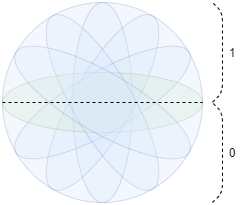Representation of Electron

An electron has the behavior of a particle and the field at the same time. And the reason is actually basic: A particle that travels in a specific orbit has the speed of light (maybe more). If we define one half of the field of that particle as 0 and the other part as 1, then our electron gets values 0 and 1 with a periodic function. This periodic function has an infinite (unknown and too big to consider) amount of frequency. In this case, it gets the value 0 and 1 at the same time. On the other hand, it starts behaving like a particle when we measure it. Because, in the end, there is always one state that can be found at time t.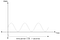State of the Electron concerning Time

But, the electrons have found a way to decrease their energy. They recognized that if they merge, the frequency of the states gets less. But, what is less than infinite? Infinity again. But just … less. They recognized that a lot of them had to merge if they needed to reach low frequencies. So, they did.

If we try to show it mathematically, it could be something like:Equation of merge of the state of two electrons

What would this look like in a graph?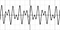The sum of two sine functions

But, Evrim, how does the frequency get less? It looks pretty much the same to me.

Okay, let’s start reading the local minima and local maxima in the graph. Starting from t=0,

0, ~0.9, 0, ~-0.47, 0, ~-0.45, …

The states of the electron get 0 or 1 if the magnitude is bigger than 0.5. Therefore, the frequency to get 0 or 1 gets less.

When the electrons merge, 0 and 1 values start appearing in longer time periods. After some time, there comes one moment where we see value 0 or value 1 for a considerable amount of time. At that moment, the way that the electrons reduce their energy starts changing. A bunch of electrons starts representing numbers such as 0, 1, 2, 3, 4, 5.3, 6.7, and so on. At this moment, the batch of electrons starts coming together to represent vectors such as [1,2,3], [5,3,7], and so on. At this moment, the reduction of energy starts being dependent on entropy. There occur vectors with size 2, then size 3, then size 4 where each size of vectors increases the entropy because increment in the size of a vector differs it from vectors having different sizes. But, there is also another way to increase entropy, vector decomposition. When the vectors get the right values, they get different orientations which increase the entropy.

So, the entropy can increase in two ways which are increment in the size of vectors or decomposition of the orientations of vectors.

So far, the electrons have found an initial way to decrease their energy, which is to merge with others. Then, they found out that they create numbers which helps them create vectors. Then, they have recognized that they can increase their entropy which will decrease their energy if they increase the size of these vectors or decompose the vectors having the same size.

What happens next? Mass. We all know the formula: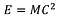Mass-energy equivalence

If the energy decreases, the mass must increase, right? It does. But how?

The long vectors start creating a considerable amount of mass because they have billions of electrons inside. So, increasing the size of a vector means we increase the mass. The bigger vectors are always more hungry to get bigger because increasing their size is easier than decomposing themselves from other same-sized vectors. Where is gravity in this equation? Gravity is the force that pulls these vectors to each other. It is a natural phenomenon that pulls the vectors to each other, and its force gets higher when the size of a vector is large because the larger vectors are likely to merge with others so that they increase their size to increase the entropy instead of decomposing themselves to increase the entropy. Our world, human beings, animals, and all other creatures in our world can be just a decomposed vectors with a size N. This actually shows that we may be unique in our universe, in the way we are. We may be actually alone in all universe.

So, there will come one moment in the universe where the vectors have the maximum size that they can get. At this point, the gravity of them will be too high that they all will merge. But, wait. If we get only one vector in the end, our entropy will collapse and go to 0 because one big vector means no information therefore no entropy. This does not make sense because our goal is to decrease our energy and to increase our entropy. So, what happens now? “Rearrangement of the vectors” happens.

Instead of merging to go to one big vector, vectors finally will start sharing their sizes for all vectors to have an equal size. When all vectors have equal sizes, they will start decomposing their orientations so that the entropy can increase. There will be an end to this decomposition as well. Will that be the absolute end of everything? Let’s think about it one more time by considering the fundamentals of this hypothesis.

Even though our electrons found a way to have a stable state for a time period, this behavior is still get controlled by a periodic function. Therefore, during this merging and decomposition process, our electrons may change their state which will cause a chain reaction where the final vectors will be affected as well. On the other hand, this periodicity can cause the perfect decomposition of the final vectors as well which will be in that state for another time period. Therefore, there will never be an end to the universe. The vectors of the universe will get into equilibrium for infinity. What about us? We will just be a part of one of those end vectors that are in equilibrium.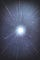Photo by Casey Horner on Unsplash

If you liked the article, please send applause, it increases the visibility.

Cheers!

AI Developer In “>”, Interested in Artificial Intelligence, Human Intelligence, Economical inequality, and all other interesting stuff.

## More from Tekin Evrim Ozmermer

AI Developer In “>”, Interested in Artificial Intelligence, Human Intelligence, Economical inequality, and all other interesting stuff.